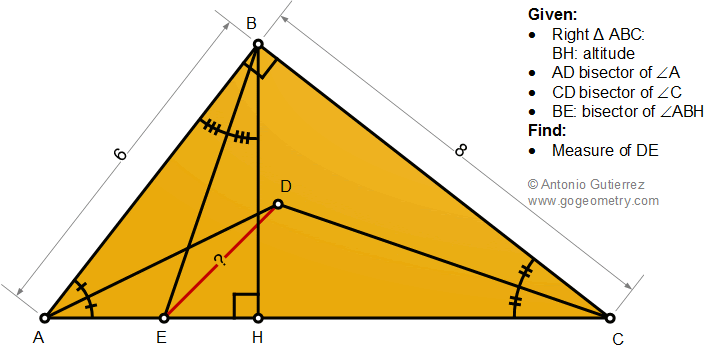## Saturday, November 28, 2020

### Geometry Problem 1487: Right Triangle, Altitude, Angle Bisectors, Measurement

Geometry Problem 1487. Post your solution in the comment box below.
Level: Mathematics Education, K-12 School, Honors Geometry, College.

Details: Click on the figure below.1.https://photos.app.goo.gl/aXxd5z7tmrZBAcbA8

Draw incircle of triangle ABC with radius r
CD meet BE at K ( see sketch)
Calculate AC= 10 , perimeter 2p= 24 , area (ABC)= 24 , r= S/p= 2
We have ∠ (ADC)=135 and ∠ (MDK)=45; ∠ (ABH)= ∠ (ACB)
∠ (BMD)= ½(∠ (BAH)+ ∠ (ABH))= 45 => CD ⊥BE
So triangle ECB is isosceles and DE=DB= r.sqrt(2)= 2.sqrt(2)

2.AB²= AH*AC or 36 =AH*10 which yields AH = 18/5 while BH = (AB*AC)/BC = 24/5. AE/EH = AB/BH or AE/AH = AB/(AB+BH) Plugging in the values as above, AH=2 and thus EC =8/ We take B as the origin and BA & BC as x-axis & y-axis resply. Then, by the division formula, E:(24/5,8/5) while D: (2.2) since the in-radius'r'of Tr. ABC =(8+6-10)/2 = 2, Finally, DE² = (24/5-2)²+ (8/5-2)² = 8 or DE = 2√2

3.ED=2V2 (V = sqrt)
Seee sketch for deatails

1.This comment has been removed by the author.

2.https://photos.app.goo.gl/TvawRXPuoCnVamtw7

3.https://photos.app.goo.gl/ZGQHdBkPirQBti2Y6

4.< DAE = 45 - C/2 = < EBD, hence ABDE is concyclic and ED = DB = y say

Now < DBC = < DBA = < DEC = 45 hence Tr.s BCD & ECD are congruent ASA
and so CE = CB = 8, whence AE = 2

Therefore EH = 6^2/10 - 2 = 8/5 & since BH = 6 X 8 / 10 = 24/5,
BE^2 = (24/5)^2 + (8/5)^2 ==> BE = (8/5).sqrt10

Now since < ADE = < ABE = < ACD = C/2, AD^2 = 2 X 10 and so AD = 2.sqrt5

Applying Ptolemy in cyclic quad ABDE, 6y + 2y = BE.AD = (8/5)sqrt10 X 2.sqrt5

Simplifying y = 2.sqrt2

Sumith Peiris
Moratuwa
Sri Lanka

5.This comment has been removed by the author.

6.Let Perpendicular from D meet AC at P
Incircle Radius DP of ABC = 2
Incircle Radius r1 of ABH = 1.2
Incircle Radius r2 of ABC = 1.6
HP=(r2-r1)=0.4 , AH=3.6, HB=4.8 , EH=1.6 (EH=HB.AE/AB)
Apply pythogorus to right-isoscelse EDP=> ED=2Sqrt(2)

1.Why HP= r2-r1 ? Please explain

Peter Tran

7.Considering using triangle notations
m(ABD)=45=> m(EBD)=45-C+C/2=45-C/2=A/2=m(DAE)
So BDEA is concyclic.
m(BED)=A/2 and m(BEH)=A+C/2=45+A/2
=>m(DEH)=m(BEH)-m(BED)=45
In-circle Radius of ABC = 2 [using r.(a+b+c)=a.c]
Let P be the foot of Perpendicular from D to AC
It can be easily found that DEP is right isosceles => DE=2Sqrt2